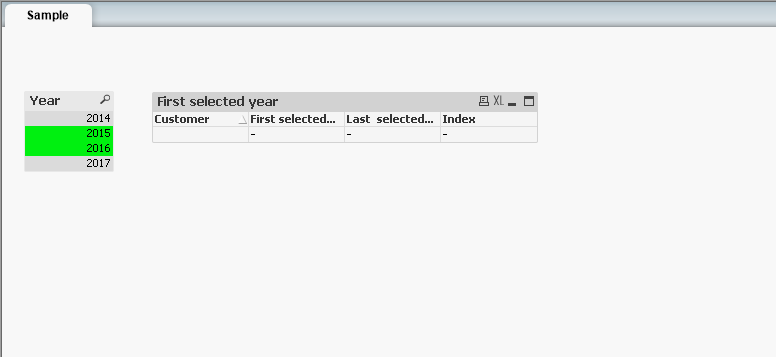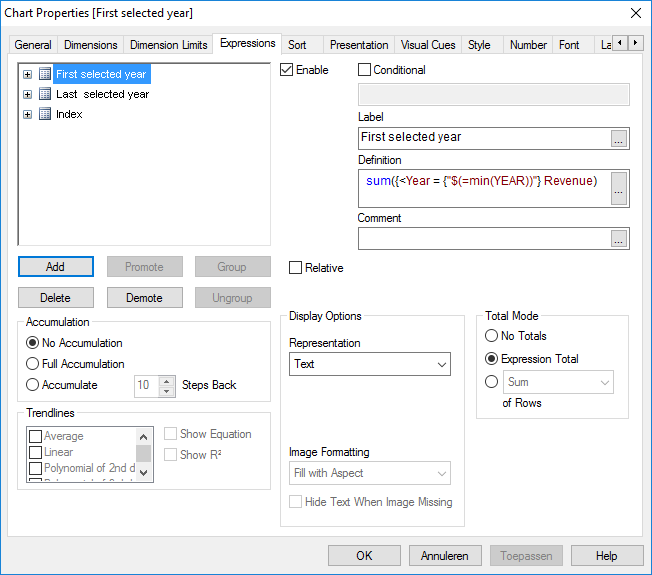# QlikView App Dev

Discussion Board for collaboration related to QlikView App Development.

Announcements
May 18th 10AM ET, Live Chat, bring your QlikView questions. REGISTER
cancel
Showing results for
Did you mean:Contributor II

## How to compare a user selection and a value?

Hi,

The user wants to compare revenue growth for two years.

Suppose a user selects 2 values of field YEAR. I need the sum of VALUES for min(YEAR) in a column and the sum of VALUES for max(YEAR) in a second column so I can compute the growth between the 2 selected years.

I was trying (using set analysis) this:

sum({<YEAR = {"\$(=min(YEAR))"} VALUE)

and

sum({<YEAR = {"\$(=max(YEAR))"} VALUE)

But this does not work. Any suggestions?

1 Solution

Accepted SolutionsMVP

You missed the closing set analysis syntax (>})

These are working now:

=Sum({<Year = {"\$(=Min(Year))"}>} Revenue)

=Sum({<Year = {"\$(=Max(Year))"}>} Revenue)7 RepliesMVP

I don't see a reason for this not working.... Can you share a sample or snapshot to show what exactly do you get and explain as to what you are looking to get?Contributor II
Author

Hi Sunny,

I don't know how to upload my sample qvw, so here some screenshots.MVP

Look here to see how a sample can be uploadedMVP

I think you are using two variation of Year (Year and YEAR).... QlikView is case sensitive and you need to make sure you are using the correct casing...

Sum({<Year = {"\$(=min(Year))"} VALUE)

or

Sum({<YEAR = {"\$(=min(YEAR))"} VALUE)Contributor II
Author

I uploaded a sample app to explain my problem.MVP

You missed the closing set analysis syntax (>})

These are working now:

=Sum({<Year = {"\$(=Min(Year))"}>} Revenue)

=Sum({<Year = {"\$(=Max(Year))"}>} Revenue)Contributor II
Author

Thanks!